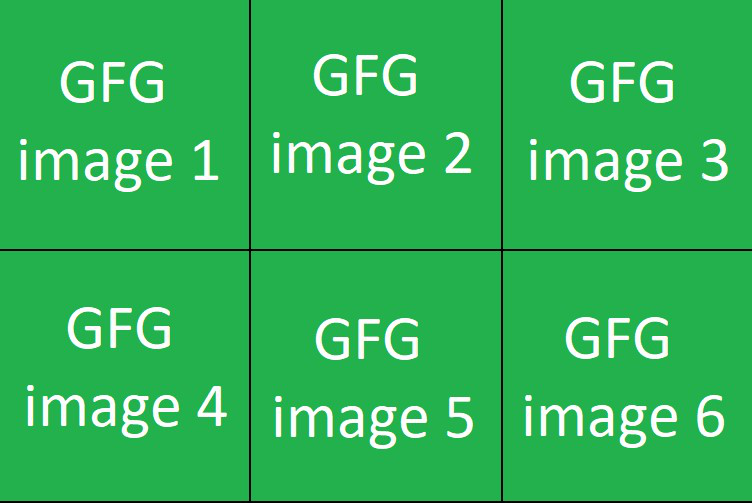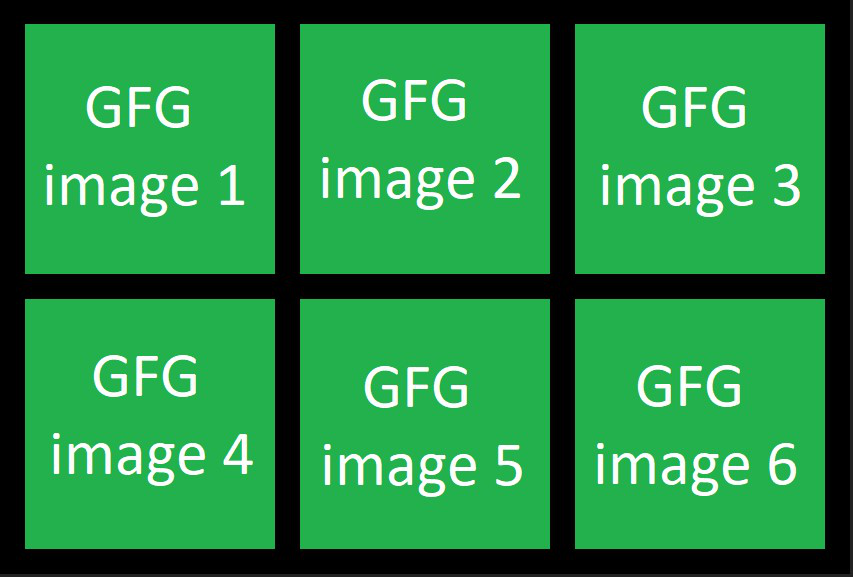GeeksforGeeks App
Open AppBrowser
Continue

# How to Make a grid of Images in PyTorch?

In this article, we are going to see How to Make a grid of Images in PyTorch. we can make a grid of images using the make_grid() function of torchvision.utils package.

## make_grid() function:

The make_grid() function accept 4D tensor with [B, C ,H ,W] shape. where B represents the batch size, C represents the number of channels, and  H, W represents the height and width respectively. The height and weight should be the same for all images. This function returns the tensor that contains a grid of input images. we can also set the number of images displayed in each row by using nrow parameter. The below syntax is used to make a grid of images in PyTorch.

Syntax: torchvision.utils.make_grid(tensor)

Parameter:

• tensor (Tensor or list) tensor of shape (B x C x H x W) or a list of images all of the same size.
• nrow (int, optional) – Number of images displayed in each row of the grid. Default: 8.

Returns: This function returns the tensor that contains a grid of input images.

Example 1:

The following example is to understand how to make a grid of images in PyTorch.

## Python3

 `# import required library``import` `torch``import` `torchvision``from` `torchvision.io ``import` `read_image``from` `torchvision.utils ``import` `make_grid`` ` `# read images from computer``a ``=` `read_image(``'a.jpg'``)``b ``=` `read_image(``'b.jpg'``)``c ``=` `read_image(``'c.jpg'``)``d ``=` `read_image(``'d.jpg'``)`` ` `# make grid from the input images``# this grid contain 4 columns and 1 row``Grid ``=` `make_grid([a, b, c, d])`` ` `# display result``img ``=` `torchvision.transforms.ToPILImage()(Grid)``img.show()`

Output:Example 2:

in the following example, we make a grid of images and set the number of images displayed in each row by using nrow Parameter.

## Python3

 `# import required library``import` `torch``import` `torchvision``from` `torchvision.io ``import` `read_image``from` `torchvision.utils ``import` `make_grid`` ` `# read images from computer``a ``=` `read_image(``'a.jpg'``)``b ``=` `read_image(``'b.jpg'``)``c ``=` `read_image(``'c.jpg'``)``d ``=` `read_image(``'d.jpg'``)``e ``=` `read_image(``'e.jpg'``)``f ``=` `read_image(``'f.jpg'``)`` ` `# make grid from the input images``# this grid contain 2 rows and 3 columns``Grid ``=` `make_grid([a, b, c, d, e, f], nrow``=``3``)`` ` `# display result``img ``=` `torchvision.transforms.ToPILImage()(Grid)``img.show()`

Output:Example 3:

In the following example, we make a grid of images and set the padding between the images.

## Python3

 `# import required library``import` `torch``import` `torchvision``from` `torchvision.io ``import` `read_image``from` `torchvision.utils ``import` `make_grid`` ` `# read images from computer``a ``=` `read_image(``'a.png'``)``b ``=` `read_image(``'b.png'``)``c ``=` `read_image(``'c.png'``)``d ``=` `read_image(``'d.png'``)``e ``=` `read_image(``'e.png'``)``f ``=` `read_image(``'f.png'``)`` ` `# make grid from the input images``# set nrow=3, and padding=25``Grid ``=` `make_grid([a, b, c, d, e, f], nrow``=``3``, padding``=``25``)`` ` `# display result``img ``=` `torchvision.transforms.ToPILImage()(Grid)``img.show()`

Output:My Personal Notes arrow_drop_up